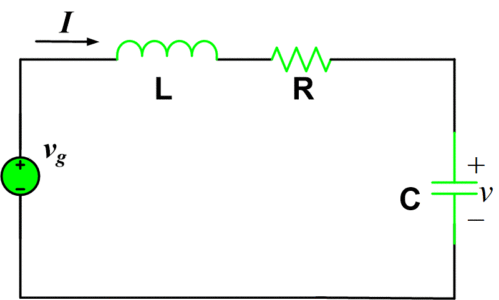# RLC Series Circuit Analysis

Want create site? Find Free WordPress Themes and plugins.

When resistance, inductance, and capacitance are connected in series, the circuit is said to be RLC Series circuit. In the RLC Series circuit shown in the figure below, the current is common to all components.

The series RLC circuit, shown in figure 1, is the dual of the parallel circuit. Therefore all the results for the parallel circuit have dual counterparts for the series circuit, which may be written down by inspection. In this section, we shall simply list these results using duality.The initial conditions will be taken as,

\begin{align} & v(0)={{V}_{0}} \\ & i(0)={{I}_{0}} \\\end{align}

The single loop equation necessary in the analysis is

$\begin{matrix} L\frac{di}{dt}+Ri+\frac{1}{C}\int\limits_{0}^{t}{idt+{{V}_{o}}={{v}_{g}}} & \cdots & (1) \\\end{matrix}$

Which is valid for t>0. The resulting characteristic equation is

$L{{s}^{2}}+Rs+\frac{1}{C}=0\text{ }\cdots \text{ (2)}$

And the natural frequencies are

Natural Frequencies\begin{align} & \begin{matrix} {{s}_{1,2}}=-\frac{R}{2L}\pm \sqrt{{{\left( \frac{R}{2L} \right)}^{2}}-\frac{1}{LC}} & \cdots & (3) \\\end{matrix} \\ & \\\end{align}

The series RLC circuit is overdamped if

$\text{ }\begin{matrix} C>\frac{4L}{{{R}^{2}}} & \cdots & (4) \\\end{matrix}$

And the response is

Over-damped Case$\begin{matrix} i={{A}_{1}}{{e}^{{{s}_{1}}t}}+{{A}_{2}}{{e}^{{{s}_{2}}t}} & \cdots & (5) \\\end{matrix}$

The circuit is critically damped if

$\text{ }\begin{matrix} C=\frac{4L}{{{R}^{2}}} & \cdots & (6) \\\end{matrix}$

In which case s1=s2=-R/2L, and the response is

Critically-damped Case$\begin{matrix} i=({{A}_{1}}+{{A}_{2}}t){{e}^{{{s}_{1}}t}} & \cdots & (7) \\\end{matrix}$

Finally, the circuit is underdamped if

$\text{ }\begin{matrix} C<\frac{4L}{{{R}^{2}}} & \cdots & (8) \\\end{matrix}$

In which case the resonant frequency is

$\begin{matrix} {{\omega }_{o}}=\frac{1}{\sqrt{LC}} & \cdots & (9) \\\end{matrix}$

A damping coefficient,

$\begin{matrix} \alpha =\frac{R}{2L} & \cdots & (10) \\\end{matrix}$

And a damped frequency,

$\begin{matrix} {{\omega }_{d}}=\sqrt{\omega _{o}^{2}-{{\alpha }^{2}}} & \cdots & (11) \\\end{matrix}$

The underdamped response is

Under-damped Case$\begin{matrix} v={{e}^{-\alpha t}}({{A}_{1}}\cos {{\omega }_{d}}t+{{A}_{2}}\sin {{\omega }_{d}}t) & \cdots & (12) \\\end{matrix}$

As an example, suppose it is required to find v, for t>0, in figure (2), given that

\begin{align} & v(0)=6V \\ & i(0)=2A \\\end{align}Fig.2: Driven series RLC Circuit

We know that

$v={{v}_{n}}+{{v}_{f}}$

Where the natural response vn contains the natural frequencies. The natural frequencies of the current I are the same as those of v because obtaining one from the other, in general, requires only Kirchhoff’s laws and the operations of addition, subtraction, multiplication by constants, integration, and differentiation. None of these operations changes the natural frequencies. Therefore, since the natural frequencies of I are easier to get (only one loop equation is required), let us obtain them. Around the loop we have

$\begin{matrix} \frac{di}{dt}+2i+5\int\limits_{0}^{t}{idt}+6=10 & \cdots & (13) \\\end{matrix}$

The characteristic equation, following differentiation, is

${{s}^{2}}+2s+5=0$

With roots

${{s}_{1,2}}=-1\pm j2$

Thus we have

$\begin{matrix} {{v}_{n}}={{e}^{-t}}({{A}_{1}}\cos 2t+{{A}_{2}}\sin 2t) & {} & {} \\\end{matrix}$

The forced response is a constant in this case and may be obtained by inspection of the circuit in the steady state. Since in the steady state, the capacitor is an open circuit and the inductor is a short circuit, if=0 and vf =10. Therefore the complete response is

$\begin{matrix} v={{e}^{-t}}({{A}_{1}}\cos 2t+{{A}_{2}}\sin 2t)+10 & {} & {} \\\end{matrix}$

From the initial voltage we have

$v(0)=6={{A}_{1}}+10$

Or A1=-4. Also, we have

$i(0)=C\frac{dV({{0}^{+}})}{dt}$

$\frac{1}{5}\frac{dv({{0}^{+}})}{dt}=i(0)=2$

$\frac{dv({{0}^{+}})}{dt}=10=2{{A}_{2}}-{{A}_{1}}$

Therefore A2=3, and we have

$\begin{matrix} v={{e}^{-t}}(-4\cos 2t+3\sin 2t)+10V & {} & {} \\\end{matrix}$